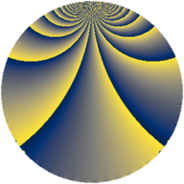# Properties

 Label 1470.2.jLevel $1470$ Weight $2$ Character orbit 1470.j Rep. character $\chi_{1470}(197,\cdot)$ Character field $\Q(\zeta_{4})$ Dimension $164$ Sturm bound $672$

# Related objects

## Defining parameters

 Level: $$N$$ $$=$$ $$1470 = 2 \cdot 3 \cdot 5 \cdot 7^{2}$$ Weight: $$k$$ $$=$$ $$2$$ Character orbit: $$[\chi]$$ $$=$$ 1470.j (of order $$4$$ and degree $$2$$) Character conductor: $$\operatorname{cond}(\chi)$$ $$=$$ $$15$$ Character field: $$\Q(i)$$ Sturm bound: $$672$$

## Dimensions

The following table gives the dimensions of various subspaces of $$M_{2}(1470, [\chi])$$.

Total New Old
Modular forms 736 164 572
Cusp forms 608 164 444
Eisenstein series 128 0 128

## Trace form

 $$164q - 4q^{3} + 4q^{6} + O(q^{10})$$ $$164q - 4q^{3} + 4q^{6} - 4q^{10} + 4q^{12} - 16q^{15} - 164q^{16} - 8q^{18} + 4q^{22} - 32q^{25} - 4q^{27} + 12q^{30} + 24q^{31} + 36q^{33} + 4q^{36} + 8q^{37} + 8q^{40} - 40q^{43} + 48q^{45} - 32q^{46} + 4q^{48} - 8q^{51} + 20q^{55} - 24q^{57} + 36q^{58} + 12q^{60} + 24q^{61} + 8q^{66} + 16q^{67} + 8q^{72} + 68q^{73} - 60q^{75} - 16q^{76} + 16q^{78} + 52q^{81} - 48q^{82} + 72q^{85} + 60q^{87} + 4q^{88} - 48q^{90} - 32q^{93} - 4q^{96} - 28q^{97} + O(q^{100})$$

## Decomposition of $$S_{2}^{\mathrm{new}}(1470, [\chi])$$ into newform subspaces

The newforms in this space have not yet been added to the LMFDB.

## Decomposition of $$S_{2}^{\mathrm{old}}(1470, [\chi])$$ into lower level spaces

$$S_{2}^{\mathrm{old}}(1470, [\chi]) \cong$$ $$S_{2}^{\mathrm{new}}(30, [\chi])$$$$^{\oplus 3}$$$$\oplus$$$$S_{2}^{\mathrm{new}}(105, [\chi])$$$$^{\oplus 4}$$$$\oplus$$$$S_{2}^{\mathrm{new}}(210, [\chi])$$$$^{\oplus 2}$$$$\oplus$$$$S_{2}^{\mathrm{new}}(735, [\chi])$$$$^{\oplus 2}$$